﻿ 基于平台调制的半解析式惯导系统高纬度工作研究

基于平台调制的半解析式惯导系统高纬度工作研究Research on High-Latitude Work of Semi-Analytical Inertial Navigation System Based on Platform Modulation

1. 引言

2. 半解析式惯导工作原理

2.1. 半解析式惯导系统平台结构及功能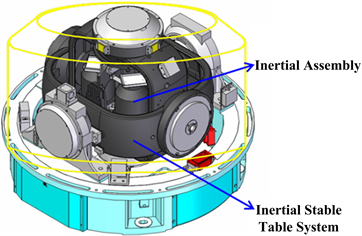Figure 1. Schematic diagram of semi-analytical inertial navigation system

2.2. 平台系统伺服回路的耦合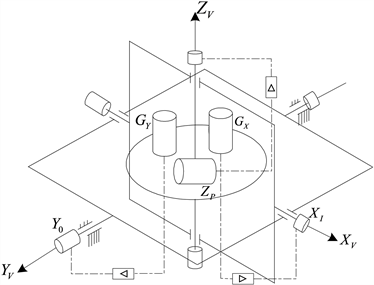Figure 2. Schematic diagram of platform frame in neutral position

$\begin{array}{l}{\omega }_{ix}={\omega }_{px}\mathrm{cos}k-{\omega }_{py}\mathrm{sin}k\\ {\omega }_{0y}={\omega }_{px}\mathrm{sin}k+{\omega }_{py}\mathrm{cos}k\end{array}\right\}$ (1)

$\begin{array}{l}{\omega }_{px}={\omega }_{ix}\mathrm{cos}k+{\omega }_{0y}\mathrm{sin}k\\ {\omega }_{py}=-{\omega }_{ix}\mathrm{sin}k+{\omega }_{0y}\mathrm{cos}k\\ {\omega }_{pz}={\omega }_{pz}\end{array}\right\}$ (2)

${C}_{P}^{I}=\left[\begin{array}{ccc}\mathrm{cos}k& \mathrm{sin}k& 0\\ -\mathrm{sin}k& \mathrm{cos}k& 0\\ 0& 0& 1\end{array}\right]$ (3)

3. 游移方位编排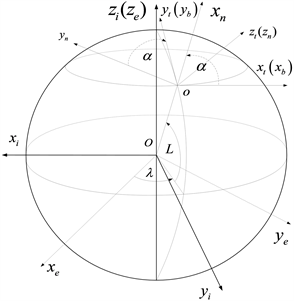Figure 3. Walking azimuth inertial navigation system coordinate system

${C}_{t}^{n}=\left[\begin{array}{ccc}\mathrm{cos}\alpha & \mathrm{sin}\alpha & 0\\ -\mathrm{sin}\alpha & \mathrm{cos}\alpha & 0\\ 0& 0& 1\end{array}\right]$ (4)

$\left[\begin{array}{c}{x}_{n}\\ {y}_{n}\\ {z}_{n}\end{array}\right]=\left[\begin{array}{c}{x}_{e}\\ {y}_{e}\\ {z}_{e}\end{array}\right]{C}_{e}^{n}$ (5)

$\begin{array}{c}{C}_{e}^{n}={C}_{t}^{n}{C}_{e}^{t}=\left[\begin{array}{ccc}\mathrm{cos}\alpha & \mathrm{sin}\alpha & 0\\ -\mathrm{sin}\alpha & \mathrm{cos}\alpha & 0\\ 0& 0& 1\end{array}\right]\left[\begin{array}{ccc}-\mathrm{sin}\lambda & \mathrm{cos}\lambda & 0\\ -\mathrm{sin}L\mathrm{cos}\lambda & -\mathrm{sin}L\mathrm{sin}\lambda & \mathrm{cos}L\\ \mathrm{cos}L\mathrm{cos}\lambda & \mathrm{sin}\lambda & \mathrm{sin}L\end{array}\right]\\ =\left[\begin{array}{ccc}-\mathrm{cos}\alpha \mathrm{sin}\lambda -\mathrm{sin}\alpha \mathrm{sin}L\mathrm{cos}\lambda & \mathrm{cos}\alpha \mathrm{cos}\lambda -\mathrm{sin}\alpha \mathrm{sin}L\mathrm{sin}\lambda & \mathrm{sin}\alpha \mathrm{cos}L\\ \mathrm{sin}\alpha \mathrm{sin}\lambda -\mathrm{cos}\alpha \mathrm{sin}L\mathrm{cos}\lambda & -\mathrm{sin}\alpha \mathrm{cos}\lambda -\mathrm{cos}\alpha \mathrm{sin}L\mathrm{sin}\lambda & \mathrm{cos}\alpha \mathrm{cos}L\\ \mathrm{cos}L\mathrm{cos}\lambda & \mathrm{cos}L\mathrm{sin}\lambda & \mathrm{sin}L\end{array}\right]\\ \text{ }=\left[\begin{array}{ccc}{C}_{11}& {C}_{12}& {C}_{13}\\ {C}_{21}& {C}_{22}& {C}_{23}\\ {C}_{31}& {C}_{32}& {C}_{33}\end{array}\right]\end{array}$ (6)

$\left\{\begin{array}{l}{\lambda }_{主}=\mathrm{arctan}\frac{{C}_{32}}{{C}_{31}}\\ L=\mathrm{arcsin}{C}_{33}\\ {\alpha }_{主}=\mathrm{arctan}\frac{{C}_{13}}{{C}_{23}}\end{array}$ (7)

${\omega }_{ie}^{n}={C}_{e}^{n}{\omega }_{ie}^{e}=\left[\begin{array}{ccc}{C}_{11}& {C}_{12}& {C}_{13}\\ {C}_{21}& {C}_{22}& {C}_{23}\\ {C}_{31}& {C}_{32}& {C}_{33}\end{array}\right]\left[\begin{array}{c}0\\ 0\\ \Omega \end{array}\right]=\left[\begin{array}{c}{C}_{13}\Omega \\ {C}_{23}\Omega \\ {C}_{33}\Omega \end{array}\right]=\left[\begin{array}{c}\Omega \mathrm{sin}\alpha \mathrm{cos}L\\ \Omega \mathrm{cos}\alpha \mathrm{cos}L\\ \Omega \mathrm{sin}L\end{array}\right]$ (8)

$\left[\begin{array}{c}{V}_{E}\\ {V}_{N}\end{array}\right]=\left[\begin{array}{cc}\mathrm{cos}\alpha & -\mathrm{sin}\alpha \\ \mathrm{sin}\alpha & \mathrm{cos}\alpha \end{array}\right]\left[\begin{array}{c}{V}_{x}\\ {V}_{y}\end{array}\right]=\left[\begin{array}{c}{V}_{x}\mathrm{cos}\alpha -{V}_{y}\mathrm{sin}\alpha \\ {V}_{x}\mathrm{sin}\alpha +{V}_{y}\mathrm{cos}\alpha \end{array}\right]$ (9)

$\begin{array}{c}{\omega }_{en}^{n}=\left[\begin{array}{ccc}\mathrm{cos}\alpha & \mathrm{sin}\alpha & 0\\ -\mathrm{sin}\alpha & \mathrm{cos}\alpha & 0\\ 0& 0& 1\end{array}\right]\left[\begin{array}{c}-\frac{{V}_{N}}{{R}_{M}}\\ \frac{{V}_{E}}{{R}_{N}}\\ 0\end{array}\right]=\left[\begin{array}{ccc}\mathrm{cos}\alpha & \mathrm{sin}\alpha & 0\\ -\mathrm{sin}\alpha & \mathrm{cos}\alpha & 0\\ 0& 0& 1\end{array}\right]\left[\begin{array}{c}-\frac{{V}_{x}\mathrm{sin}\alpha +{V}_{y}\mathrm{cos}\alpha }{{R}_{M}}\\ \frac{{V}_{x}\mathrm{cos}\alpha -{V}_{y}\mathrm{sin}\alpha }{{R}_{N}}\\ 0\end{array}\right]\\ =\left[\begin{array}{c}-{V}_{x}\mathrm{sin}\alpha \mathrm{cos}\alpha \left(\frac{1}{{R}_{M}}-\frac{1}{{R}_{N}}\right)-{V}_{y}\left(\frac{{\mathrm{cos}}^{2}\alpha }{{R}_{M}}-\frac{{\mathrm{sin}}^{2}\alpha }{{R}_{N}}\right)\\ {V}_{x}\left(\frac{{\mathrm{cos}}^{2}\alpha }{{R}_{M}}+\frac{{\mathrm{sin}}^{2}\alpha }{{R}_{N}}\right)+{V}_{y}\mathrm{sin}\alpha \mathrm{cos}\alpha \left(\frac{1}{{R}_{M}}-\frac{1}{{R}_{N}}\right)\\ 0\end{array}\right]\end{array}$ (10)

${\omega }_{in}^{n}={\omega }_{ie}^{n}+{\omega }_{en}^{n}\text{ }=\left[\begin{array}{c}\Omega \mathrm{sin}\alpha \mathrm{cos}L-{V}_{x}\mathrm{sin}\alpha \mathrm{cos}\alpha \left(\frac{1}{{R}_{M}}-\frac{1}{{R}_{N}}\right)-{V}_{y}\left(\frac{{\mathrm{cos}}^{2}\alpha }{{R}_{M}}-\frac{{\mathrm{sin}}^{2}\alpha }{{R}_{N}}\right)\\ \Omega \mathrm{cos}\alpha \mathrm{cos}L+{V}_{x}\left(\frac{{\mathrm{cos}}^{2}\alpha }{{R}_{M}}+\frac{{\mathrm{sin}}^{2}\alpha }{{R}_{N}}\right)+{V}_{y}\mathrm{sin}\alpha \mathrm{cos}\alpha \left(\frac{1}{{R}_{M}}-\frac{1}{{R}_{N}}\right)\\ \Omega \mathrm{sin}L\end{array}\right]=\left[\begin{array}{c}{\omega }_{inx}^{n}\\ {\omega }_{iny}^{n}\\ {\omega }_{inz}^{n}\end{array}\right]$ (11)

$\left[\begin{array}{ccc}{\stackrel{˙}{C}}_{11}& {\stackrel{˙}{C}}_{12}& {\stackrel{˙}{C}}_{13}\\ {\stackrel{˙}{C}}_{21}& {\stackrel{˙}{C}}_{22}& {\stackrel{˙}{C}}_{23}\\ {\stackrel{˙}{C}}_{31}& {\stackrel{˙}{C}}_{32}& {\stackrel{˙}{C}}_{33}\end{array}\right]=\left[\begin{array}{ccc}0& 0& -{\omega }_{eny}^{n}\\ 0& 0& {\omega }_{enx}^{n}\\ {\omega }_{eny}^{n}& -{\omega }_{enx}^{n}& 0\end{array}\right]\left[\begin{array}{ccc}{C}_{11}& {C}_{12}& {C}_{13}\\ {C}_{21}& {C}_{22}& {C}_{23}\\ {C}_{31}& {C}_{32}& {C}_{33}\end{array}\right]$ (12)

$\stackrel{˙}{V}={f}^{n}-\left(2{\omega }_{ie}^{n}+{\omega }_{en}^{n}\right)×V+{g}^{n}$ (13)

${\psi }_{真}=\psi +\alpha$ (17)

$\stackrel{˙}{\alpha }=\Omega \mathrm{sin}L-\left(\Omega \mathrm{sin}L+\frac{{V}_{E}}{{R}_{N}}\mathrm{tan}L\right)=-\frac{{V}_{E}}{{R}_{N}}\mathrm{tan}L$ (18)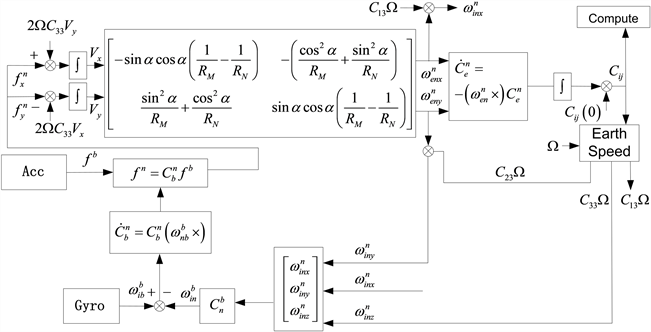Figure 4. Mechanical layout block diagram of walking azimuth inertial navigation system

4. 基于惯性平台调制的高纬度工作方案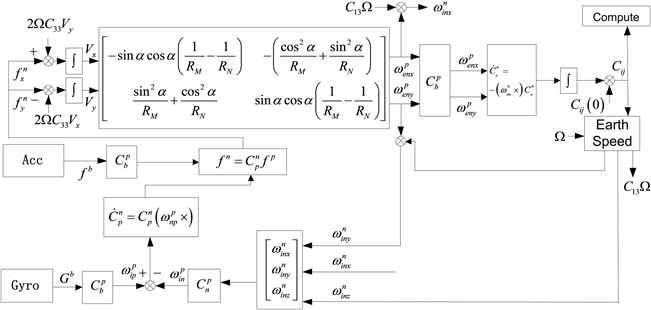Figure 5. Semi-analytical high latitude mechanical arrangement of inertial navigation with rotation modulation of inertial platform

$k\left(t\right)={k}_{0}-{\int }_{0}^{t}\left(\Omega \mathrm{sin}\phi +{\omega }_{TZ}\mathrm{sin}\phi +\frac{{V}_{x}}{R}\mathrm{tan}\phi \right)\text{d}t$ (19)

$\left[\begin{array}{c}{\omega }_{kpx}\\ {\omega }_{kpy}\end{array}\right]=\left[\begin{array}{cc}\mathrm{cos}k& \mathrm{sin}k\\ -\mathrm{sin}k& \mathrm{cos}k\end{array}\right]\left[\begin{array}{c}{\omega }_{cx}\\ {\omega }_{cy}\end{array}\right]$ (20)

$\delta {\stackrel{˙}{C}}_{e}^{p}={\Omega }_{pe}\cdot \delta {C}_{e}^{p}$ (21)

${\Omega }_{pe}=-{\Omega }_{ep}=\left[\begin{array}{ccc}0& 0& -{\omega }_{epy}\\ 0& 0& {\omega }_{epx}\\ {\omega }_{epy}& -{\omega }_{epx}& 0\end{array}\right]$ (22)

${\omega }_{ep}$ 有如下关系：

$\left[\begin{array}{c}{\omega }_{epx}\\ {\omega }_{epy}\end{array}\right]=\left[\begin{array}{cc}-\frac{1}{{R}_{px}}& \frac{1}{{\xi }_{k}}\\ -\frac{1}{{\xi }_{k}}& \frac{1}{{R}_{py}}\end{array}\right]\left[\begin{array}{c}{V}_{px}\\ {V}_{py}\end{array}\right]$ (23)

$\left\{\begin{array}{l}\frac{1}{{R}_{px}}=\frac{{\mathrm{cos}}^{2}k}{{R}_{M}}+\frac{{\mathrm{sin}}^{2}k}{{R}_{N}}\\ \frac{1}{{R}_{py}}=\frac{{\mathrm{sin}}^{2}k}{{R}_{M}}+\frac{{\mathrm{cos}}^{2}k}{{R}_{N}}\\ \frac{1}{{\xi }_{k}}=\left(\frac{1}{{R}_{N}}-\frac{1}{{R}_{M}}\right)\mathrm{sin}k\mathrm{cos}k\end{array}$ (24)

$\left\{\begin{array}{l}{\omega }_{epx\left(t\right)}={C}_{13}\Omega +{\omega }_{epx\left(t-1\right)}\\ {\omega }_{epy\left(t\right)}={C}_{23}\Omega +{\omega }_{eyx\left(t-1\right)}\end{array}$ (25)

$\left\{\begin{array}{l}{\stackrel{˙}{V}}_{px}={A}_{px}+\Omega {C}_{33}{V}_{py}\\ {\stackrel{˙}{V}}_{py}={A}_{py}-\Omega {C}_{33}{V}_{px}\end{array}$ (26)

5. 格网导航

${C}_{g}^{G}=\left[\begin{array}{ccc}\mathrm{cos}\sigma & -\mathrm{sin}\sigma & 0\\ \mathrm{sin}\sigma & \mathrm{cos}\sigma & 0\\ 0& 0& 1\end{array}\right]$ (27)

$\sigma$ ：格网角。

${C}_{b}^{G}={C}_{g}^{G}{C}_{b}^{n}=\left[\begin{array}{ccc}×& \mathrm{sin}\left(\psi -\sigma \right)\mathrm{cos}\theta & ×\\ ×& \mathrm{cos}\left(\psi -\sigma \right)\mathrm{cos}\theta & ×\\ -\mathrm{sin}\gamma \mathrm{cos}\theta & \mathrm{sin}\theta & \mathrm{cos}\gamma \mathrm{cos}\theta \end{array}\right]$ (28)

$\psi$ ：地理航向；

$\theta$ ：纵摇角；

$\gamma$ ：横摇角；

${\psi }_{格}=\psi -\sigma$ (29)

6. 极区导航输出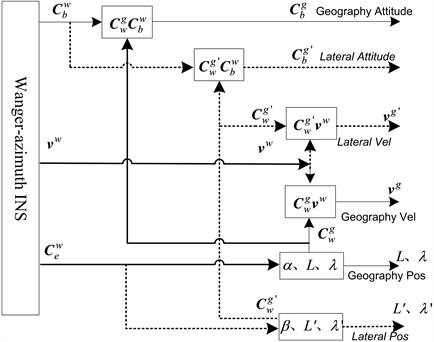Figure 6. Polar navigation mechanical layout7. 结论

 Yao, Y. (2016) Transverse Navigation under the Ellipsoidal Earth Model and Its Performance in Both Polar and Non-Polar Areas. Journal of Navigation, 69, 335-352.
https://doi.org/10.1017/S0373463315000715

 Li, Q., Ben, Y.Y. and Yu, F. (2015) System Reset of Transversal Strapdown INS for Ship in Polar Region. Measurement, 60, 247-257.
https://doi.org/10.1016/j.measurement.2014.10.020

 Goshen-Meskin, D. and Bar-Itzhack, I.Y. (1992) Observability Analysis of Piece-Wise Constant Systems. I. Theory. IEEE Transactions on Aerospace and Electronic Systems, 28, 1056-1067.
https://doi.org/10.1109/7.165367

 周凌峰, 赵小明, 翁海娜, 等. 基于惯导误差的多波束测深精度分析[J]. 中国惯性技术学报, 2013, 21(6): 758-762.

 Xiong, Z., Chen, H.M. and Yu, F. (2010) Research of Airborne INS/STAR Integrated Algorithm Coupled with Position Error. Journal of Astronoutis, 31, 2683-2690.

 高钟毓. 惯性导航系统技术[M]. 北京: 清华大学出版社, 2012: 203-230.

 Chen, W.X., Wang, L., Zheng, T., et al. (2017) Research on Calibration Method of Installation Error of Star Sensor in Inertial Platform. Navigation and Control, 16, 71-75.

 Greenaway, K.R. and Gates, M.D. (2009) Polar Air Navigation—A Record. Art Bookbindery, Canada.

 Kong, X.-W., Guo, M.-F. and Dong, J.-X. (2010) Observability and Maneuvering for Rapid Transfer Alignment of Strapdown Inertial Navigation System. Journal Tsinghua University (Sci & Tech), 50, 232-236.

 杨波, 王跃刚, 单斌, 等. 长航时环境下高精度组合导航方法研究与仿真[J]. 宇航学报, 2011, 32(5): 1054-1059.

Top